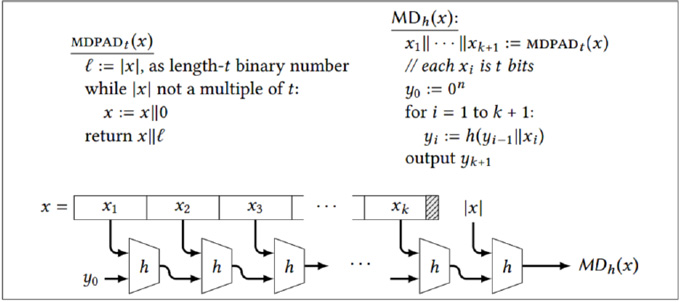# 12.3: Merkle-Damgård Construction

Constructing a hash function seems like a challenging task, especially given that it must accept strings of arbitrary length as input. In this section, we’ll see one approach for constructing hash functions, called the Merkle-Damgård construction.

Instead of a full-fledged hash function, imagine that we had a collision-resistant function (family) whose inputs were of a single fixed length, but longer than its outputs. In other words, suppose we had a family ℋ of functions h : {0,1}n+t → {0,1}n, where t > 0. We call such an h a compression function. This is not compression in the usual sense of data compression — we are not concerned about recovering the input from the output. We call it a compression function because it “compresses” its input by t bits (analogous to how a pseudorandom generator “stretches” its input by some amount).

We can apply the standard definition of collision-resistance to a family of compression functions, by restricting our interest to inputs of length exactly n + t. The functions in the family are not defined for any other input length.

The following construction is one way to extend a compression function into a full-edged hash function accepting arbitrary-length inputs:

Construction 12.4: Merkle-Damgård

Let h : {0,1}n + t → {0,1}n be a compression function. Then the Merkle-Damgård transformation of h is MDh : {0,1} → {0,1}n, where:The idea of the Merkle-Damgård construction is to split the input x into blocks of size t. The end of the string is filled out with 0s if necessary. A final block called the “padding block” is added, which encodes the (original) length of x in binary.

We are presenting a simplified version, in which MDh accepts inputs whose maximum length is 2t − 1 bits (the length of the input must fit into t bits). By using multiple padding blocks (when necessary) and a suitable encoding of the original string length, the construction can be made to accomodate inputs of arbitrary length (see the exercises).

The value y0 is called the initialization vector (IV), and it is a hard-coded part of the algorithm. In practice, a more “random-looking” value is used as the initialization vector. Or one can think of the Merkle-Damgård construction as defining a family of hash functions, corresponding to the different choices of IV.

Claim 12.5

Letbe a family of compression functions, and define MD = {MDh | h∈ ℋ} (a family of hash functions). Ifis collision-resistant, then so is MD.

Proof

While the proof can be carried out in the style of our library-based security definitions, it’s actually much easier to simply show the following: given any collision under MDh, we can efficiently find a collision under h. This means that any successful adversary violating the collision-resistance of MD can be transformed into a successful adversary violating the collision resistance of ℋ. So if ℋ is collision-resistant, then so is MD.

Suppose that x,x’ are a collision under MDh. Define the values x1,. . . ,xk + 1 and y1,. . . ,yk + 1 as in the computation of MDh (x). Similarly, define x’1,. . . ,x’k’+1 and y’1,. . . ,y’k’ + 1 as in the computation of MDh (x’). Note that, in general, k may not equal k’.

Recall that:

MDh (x) = yk + 1 = h(ykxk + 1)

MDh (x’) = y’k’ + 1 = h(y’k’x’k’ + 1)

Since we are assuming MDh(x) = MDh(x’), we have yk + 1 = y’k’ + 1. We consider two cases:

Case 1: If |x| ≠ |x’|, then the padding blocks xk + 1 and x’k’ + 1 which encode |x| and |x’| are not equal. Hence we have ykxk + 1y’k’x’k’ + 1, so ykxk + 1 and y’k’x’k’ + 1 are a collision under h and we are done.

Case 2: : If |x| = |x’|, then x
and x’ are broken into the same number of blocks, so k = k’. Let us work backwards from the final step in the computations of MDh (x) and MDh(x’). We know that:If ykxk + 1 and y’kx’k + 1 are not equal, then they are a collision under h and we are done. Otherwise, we can apply the same logic again to yk and y’k, which are equal by our assumption.

More generally, if yi = y’i, then either yi − 1xi and y’i − 1x’i are a collision under h (and we say we are “lucky”), or else yi − 1 = y’i − 1 (and we say we are “unlucky”). We start with the premise that yk = y’k. Can we ever get “unlucky” every time, and not encounter a collision when propagating this logic back through the computations of MDh(x) and MDh(x’)? The answer is no, because encountering the unlucky case every time would imply that xi = x’i for all i. That is, x = x’. But this contradicts our original assumption that x ≠ x’. Hence we must encounter some “lucky” case and therefore a collision in h.